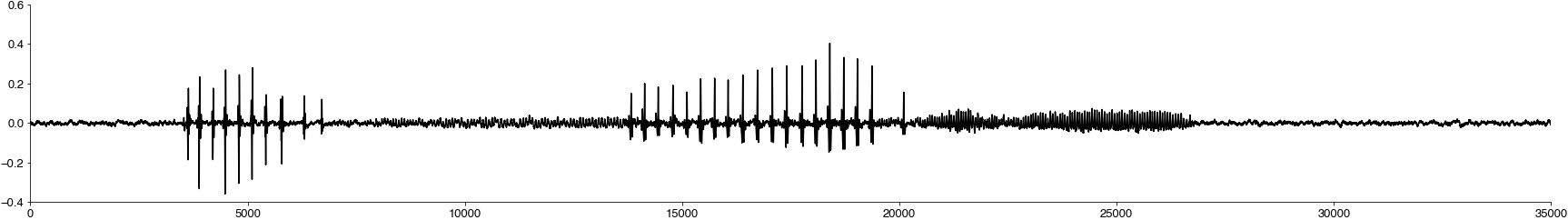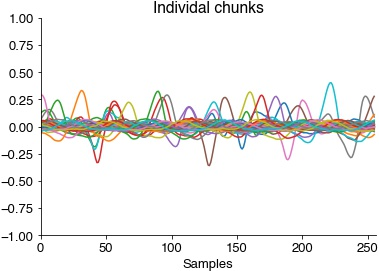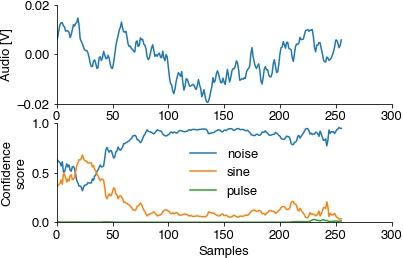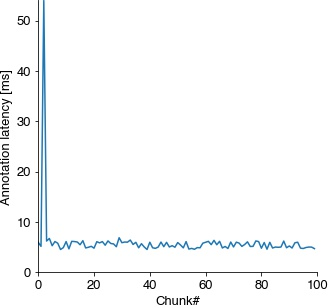# Annotate audio in realtime#

## Setup#

Test the latency of realtime annotations on a GPU (if you have one) or a CPU.

Disabling eager execution is crucial for low latency annotation - eager execution increases latencies 6-fold, from 5 ms to 30 ms.

```import os
import tensorflow as tf
USE_GPU = False  #
# disable existing GPUs if testing CPU
os.environ['CUDA_VISIBLE_DEVICES'] = '0' if USE_GPU else '-1'
# disabling eager execution in tensorflow speeds up annotations by ~6x
try:
from tensorflow.python.framework.ops import disable_eager_execution
disable_eager_execution()

tf.compat.v1.disable_eager_execution()

physical_devices = tf.config.list_physical_devices('GPU')
print('GPUs:', physical_devices)
physical_devices = tf.config.list_physical_devices('CPU')
print('CPUs:', physical_devices)
except Exception as e:
print(e)
```
```GPUs: []
CPUs: [PhysicalDevice(name='/physical_device:CPU:0', device_type='CPU')]
```
```%config InlineBackend.figure_format = 'jpg'  # smaller mem footprint for page

import numpy as np
import das.utils
import das.predict
import scipy.signal
import matplotlib.pyplot as plt
import scipy.io.wavfile
import time
import subprocess

plt.style.use('ncb.mplstyle')
```

## Load the model and runtime parameters#

```model_filename = 'models/dmel_single_rt/20200430_201821'

pulse_index = params['class_names'].index('pulse')
sine_index = params['class_names'].index('sine')

samplerate = params['samplerate_x_Hz']
interval = params['nb_hist'] / samplerate

print(f"Model expects input shape {model.input_shape} samples and {model.input_shape} channel(s).")
```
```/Users/clemens10/miniconda3/lib/python3.7/site-packages/tensorflow/python/keras/layers/core.py:1061: UserWarning: dss.tcn.tcn is not loaded, but a Lambda layer uses it. It may cause errors.
, UserWarning)
```
```Model expects input shape 256 samples and 1 channel(s).
```

Samplerates of the recording and of the data the model was trained with should match. Otherwise resample the recording to match the model using `scipy.signal.resample_poly(x=recording, up=int(recording_samplerate), down=int(samplerate), axis=0)`

```recording_samplerate, recording = scipy.io.wavfile.read('dat/dmel_song_rt.wav')
recording = np.atleast_2d(recording).T

plt.figure(figsize=(30, 4))
plt.plot(recording[:100_000], c='k')  # plot the first 10 seconds
plt.show()

print(f"Sample rate of the recording ({recording_samplerate}Hz), sample rate expected by the model ({samplerate}Hz). Both should match.")
``````Sample rate of the recording (10000Hz), sample rate expected by the model (10000Hz). Both should match.
```

## Define a virtual microphone for testing#

To demonstrate real-time inference, we create a virtual microphone that returns chunk of audio data in real time. The microphone takes a full recording and returns chunks of data at a rate determined by the chunk duration. In a real experimental setup, this would be a function that runs the data acquisition or a multi-processing queue that gets fed by the acquisition system.

```def virtual_microphone(data: np.array, interval: float, samplerate: float, block: bool = True):

RUN = True
current_position = 0
t1 = time.time() - 0.025
t0 = time.time()
while RUN:
current_position += interval * samplerate
start_sample = int(current_position)
end_sample = int(current_position + interval * samplerate)
chunk = data[start_sample:end_sample]
if block:
while (t1-t0)<interval:  # wait til interval has elapsed
time.sleep(0.001)
t1 = time.time()
if end_sample >= data.shape:
RUN = False
yield None
else:
yield chunk
t0 = time.time()

mike = virtual_microphone(recording, interval, samplerate, block=True)
rec = next(mike)
print(f"Microphone produces {rec.shape} samples and {rec.shape} channel(s) per chunk.")

print('Consuming and plotting 100 chunks...')
recording_latencies = []
for _ in range(100):
with das.utils.Timer() as t:
rec = next(mike)
recording_latencies.append(t.elapsed * 1000)
plt.plot(rec)
plt.ylim(-1, 1)
plt.title('Individal chunks')
plt.xlabel('Samples')
plt.xlim(0, rec.shape)
plt.show()
print(f"Takes {np.median(recording_latencies):1.1f} ms to return {rec.shape / samplerate * 1000:1.1f} ms of audio data.")
```
```Microphone produces 256 samples and 1 channel(s) per chunk.
Consuming and plotting 100 chunks...
``````Takes 26.0 ms to return 25.6 ms of audio data.
```

## Test real-time annotation#

Test the model and a simple song detection routine with a chunk that contains song. A specific song type is detect if the corresponding confidence exceeds 0.7 during a chunk.

```def report_song(prediction, pulse_index, sine_index):
report = 'pulse? ' + ('yes' if np.max(out[:, pulse_index]) > 0.7 else ' no')
report += ' sine? ' + ('yes' if np.max(out[:, sine_index]) > 0.7 else ' no')
return report

# consume a couple of chunks until there is song
for _ in range(9):
d = next(mike)

d = d[np.newaxis, ...]
out = model.predict(d, verbose=0)

plt.subplot(211)
plt.plot(d[0, ...])
plt.ylabel('Audio [V]')
plt.subplot(212)
plt.plot(out)
plt.ylabel('Confidence\nscore')
plt.xlabel('Samples')
plt.legend(params['class_names'])
```
```/Users/clemens10/miniconda3/lib/python3.7/site-packages/tensorflow/python/keras/engine/training.py:2426: UserWarning: `Model.state_updates` will be removed in a future version. This property should not be used in TensorFlow 2.0, as `updates` are applied automatically.
warnings.warn('`Model.state_updates` will be removed in a future version. '
```
```<matplotlib.legend.Legend at 0x7ffdec78f4d0>
```## Run annotation#

Run the virtual microphone for 100 chunks to estimate annotation latencies.

```latencies = []
runs = 0
mike = virtual_microphone(recording, interval, samplerate, block=True)

while runs < 100:
runs += 1
with das.utils.Timer() as dt:
d = next(mike)  # get a new chunk
d = d[np.newaxis, ...]
out = model.predict(d, verbose=0)  # run inference on the chunk
# detect pulse and sine
result = report_song(out[0, ...], pulse_index, sine_index)
# print(result)
latencies.append(dt.elapsed * 1000)
```

## Evaluate latencies#

Timing on GPUs can be more variable than on CPUs since a major contributor to latency is the transfer of data from/to the GPU.

Subtract the recording latency to get an estimate of the annotation latency.

```annotation_latencies = np.array(latencies) - np.median(recording_latencies)
print('Annotation latencies')
print(f'  5, 50, 95 percentiles: {np.percentile(annotation_latencies, [5, 50, 95])} ms')
print(f'  min {np.min(annotation_latencies):1.2f} ms, max {np.max(annotation_latencies):1.2f} ms')

plt.figure(figsize=(5, 5))
plt.plot(np.array(annotation_latencies))
plt.ylabel('Annotation latency [ms]')
plt.xlabel('Chunk#')
plt.ylim(0, np.max(annotation_latencies))
plt.show()
```
```Annotation latencies
5, 50, 95 percentiles: [4.6493622 5.443717  6.2767092] ms
min 4.53 ms, max 54.00 ms
```